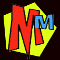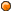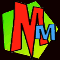How to solve quadratic equations by... COMPLETING THE SQUARE

The Phantom X tells you all about quadratic equations. These are equations which have a squared term in them such as x2 +3x -4 = 0 . The odd bit is that these equations usually have TWO different answers that work! (With the equation x2 +3x -4 = 0, x can either = 1 or -4.)The QUADRATIC FORMULA (hidden behind a secret panel) Simple quadratics just have whole numbers as the answer, and the book shows you how to solve them. Really tough quadratics often have decimals as the answer (or even imaginary answers!) and the book also shows you how to solve these using the amazing QUADRATIC FORMULA. However there is another rather neat way of solving tough quadratics without using the formula. It's called "Completing The Square". Sadly it's a bit old fashioned so not many people remember how to do it, which is a shame because it's rather a neat trick!

 Suppose you need to solve this grim looking quadratic equation (and we're warning you, the answers are not whole numbers!): x2 + 5x - 9 = 0 First move the constant across. Here we do it by adding 9 to both sides: x2 + 5x = 9Now we're going to make a new equation by playing with the Left Hand Side (or LHS). We'll ignore the RHS for a moment. Follow these instructions exactly. 1/ divide the LHS by x . In this case we get x + 5 2/ divide the number by 2 (don't divide the "x"). Now we get x +2.5 3/ put both terms in a bracket, square it and then multiply it all out (x + 2.5)2           = (x +2.5)(x +2.5)           = x2 + 2.5x + 2.5x + 6.25           = x2 + 5x + 6.25Here comes the coolest part of the whole operation. Because we know that x2 + 5x =9 (look back a few lines, you'll find it written there!) we can swap the x2 +5x on the RHS for 9. Now we've got our new equation (x +2.5)2 = 9 + 6.25 Then a quick little sum gives us... (x + 2·5)2 = 15·25 We now take the square root of both sides, and the clever bit is that square roots can be + or - Here we get: x + 2·5 = + sqrt(15.25) OR         x + 2.5 = - sqrt(15.25) Grab a calculator to work out the square root... x + 2·5 = + 3.905 OR         x + 2.5 = - 3.905 And then when you take away the 2·5 from both sides you get the two solutions x = +3·905 -2.5 = 1·405 OR x = -3·905 -2.5 = - 6·405 And that's it! The two answers to x2 + 5x - 9 = 0 are x = + 1.405 OR x = - 6.405Here's another one just to make sure you've got it. To make it more exciting this one has an x2 coefficient!

 We're going to solve this little baby... 3x2 - 11x - 8 = 0 Move the constant over 3x2 - 11x = 8 Before we go on, we divide everything by the x2 coefficient because we want the x2 by itself x2 - 11x/3 = 8/3Here's where we start making our new equation... Make the LHS into a square using steps 1,2 and 3 as before. (So divide through by x, then divide the constant by 2, then put the answer in a bracket and square it.) (x - 11/6)2 Multiply the square out (x - 11/6)2 = x2 - 11x/6 - 11x/6 +121/36                   = x2 - 11x/3 + 121/36 Now it's time to play the cool little trick! We know frombefore that x2 - 11x/3 = 8/3 so we put this into the RHS... ... and here's the new equation: (x - 11/6)2 = 8/3 + 121/36 At this point we'll make everything into decimals: (x - 1.833)2 = 2.667 + 3.361 = 6.028 Take square roots of both sides x - 1.833 = + sqrt(6.028) OR x - 1.833 = - sqrt(6.028) Get the calculator and work out the square root... x - 1.833 = + 2.455 OR x - 1.833 = - 2.455 If we add 1.833 to both sides we get you get the two answers: x = +1·833 + 2·455 = 4·288 OR x = +1·833 -2.455 = - 0·622 So if 3x2 - 11x - 8 = 0 then x = 4·288 OR x = - 0·622

And if you don't believe it, you can always check the answers on our QUADRATIC SOLVER!To THE PHANTOM X (the algebra book)

To the Research Lab